# Mathematics Library

Closed - Full Hours /

## Recommended Books

On this page you will find recommended readings for Math Majors. The books were selected by faculty and graduate students in the Department of Mathematics and by tutors and staff of the Math Support Center. Use the ‘filter by’ option to narrow down the selection of books to just the topic you want to explore.A concrete introduction to higher algebra (12656388)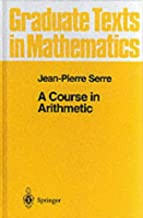A Course in Arithmetic (12622912)A First Course in Abstract Algebra (4519622)A friendly introduction to number theory (3157707)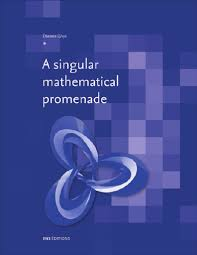African American firsts in science and technology (3484608)Algebraic curves: an introduction to algebraic geometry (1601414)Algebraic theory of numbers (11389345)Amusements in Mathematics (12129344)An illustrated theory of numbers (10061074)An Imaginary Tale: The Story of i (9830275)An introduction to the theory of numbers (6378112)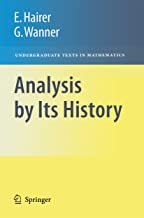Analysis by Its History (12001424)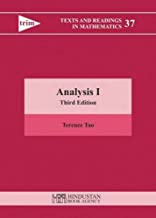Analysis I (13056775)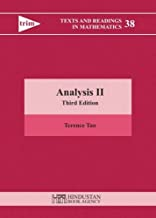Analysis II (13056777)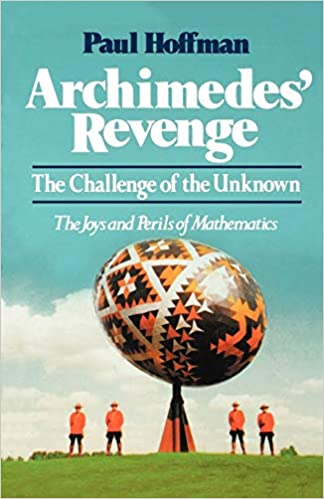Archimedes' revenge: the joys and perils of mathematics (1536031)Arithmetic (10000288)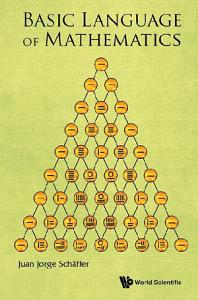Basic language of mathematics (8692746)Birth of a theorem: a mathematical adventure (8894962)Bridges to infinity: the human side of mathematics (898371)Calculus gems: brief lives and memorable mathematics (13084791)Calculus with applications (12401609)